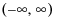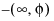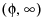• Let b estimate the parameters β so that we have b distributed as N(β,V).
 • Let x be the regressor values of interest, with the ith value to be estimated.
 • Let y be the response value.
We desire a confidence region on the value of x[i] such that β’x = y with all other values of x given.
where the parenthesized subscript (i) indicates that the ith component is omitted. A confidence interval can be formed from the relation
where t is the t value for the specified confidence level.
 • an interval of the form (φ1, φ2), where φ1 < φ2
 •
 • the entire real line,• only one oforNote: The Fit Y by X logistic platform and the Fit Model Nominal Logistic personalities use t values when computing confidence intervals for inverse prediction. The Fit Model Generalized Linear Model personality, as well as PROC PROBIT in SAS/STAT, use z values, which give different results.